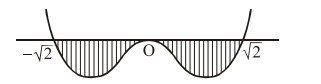# Solve this following`
Question:

Let $I=\int_{a}^{b}\left(x^{4}-2 x^{2}\right) d x$. If I is minimum then

the ordered pair (a, b) is :

1. $(-\sqrt{2}, 0)$

2. $(-\sqrt{2}, \sqrt{2})$

3. $(0, \sqrt{2})$

4. $(\sqrt{2},-\sqrt{2})$

Correct Option: , 2

Solution:

Let $f(x)=x^{2}\left(x^{2}-2\right)$As long as $f(x)$ lie below the $x$-axis, definite integral will remain negative,

so correct value of $(a, b)$ is $(-\sqrt{2}, \sqrt{2})$ for minimum of I